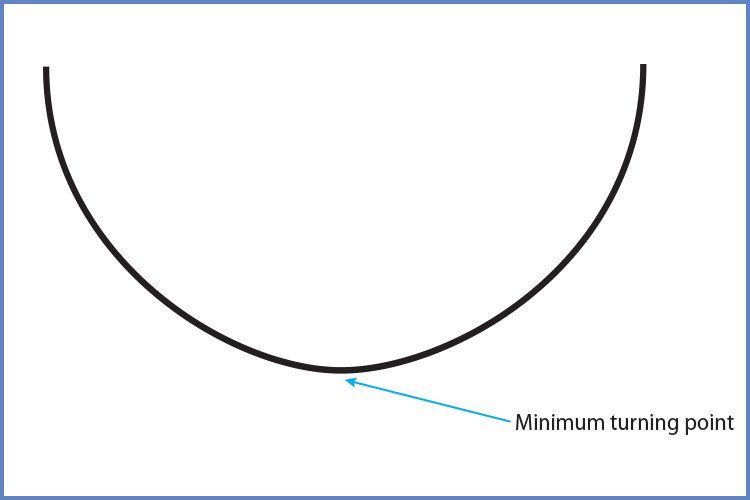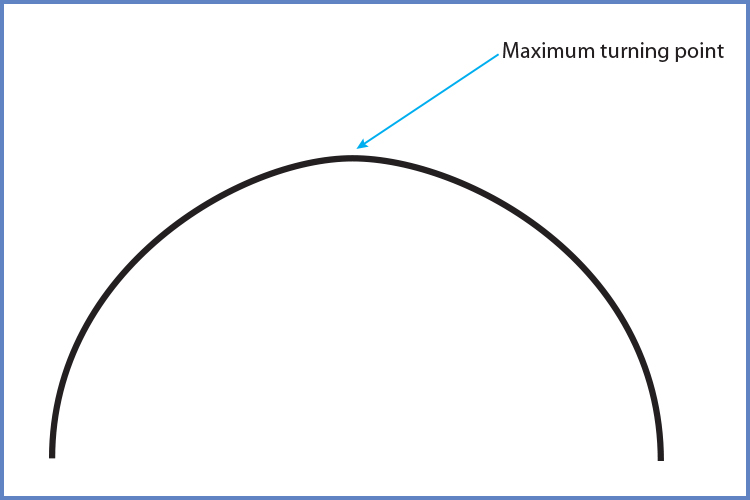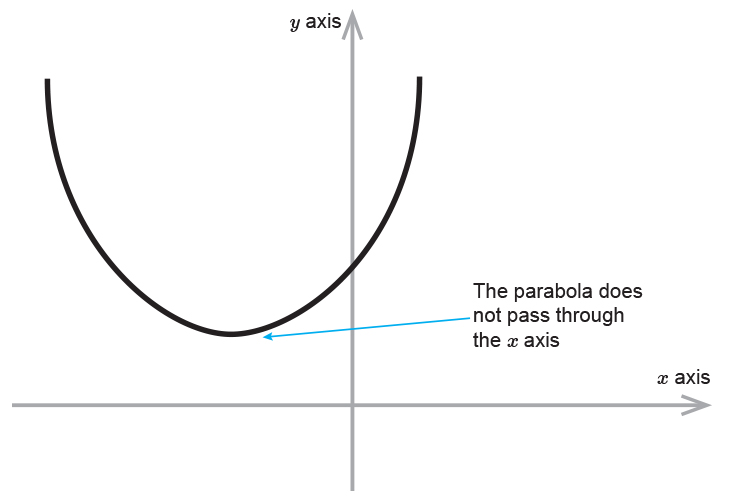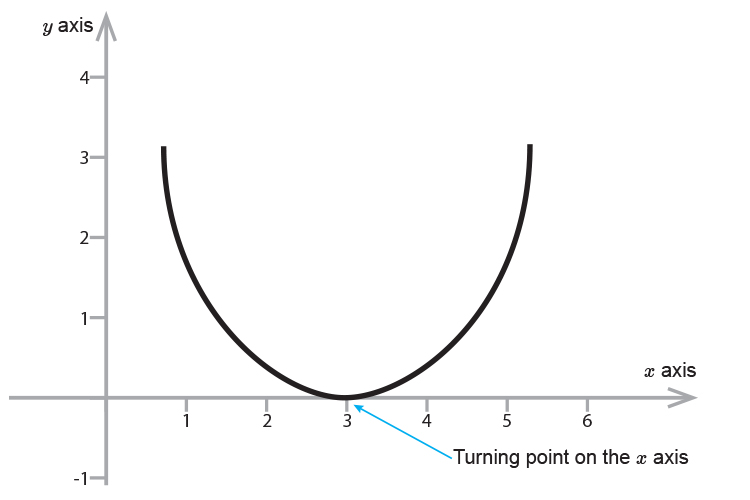# Interpreting parabola formulas

The general formula for a parabola is ax^2+bx+c=y

## Positive and negative x^2 coefficients

If x^2 is positive then your parabola will look like this:If x^2 is negative then your parabola will look like this:## Can't find the root

NOTE:

See later on finding the solution is finding the root or roots which is where the curve passes the x  axis.

If in finding the solution to ax^2+bx+c=y

x=+-sqrt(-12)     (for example)

It's not possible to find the square root of a negative number.

(To prove this put 3 then minus 6 into your calculator and then press the root button. You will get an error message.)Your parabola will look something like the diagram below, meaning that the curve doesn't cross the x  axis.NOTE:

x=+-sqrt(-12)  is called "Quadratics and complex numbers" and if you found this in an exam at this stage you've done something wrong.

## Only one root value

If in finding the solution to:

ax^2+bx+c=y

x=3  (for example)

And NOT   x=-1\ \ \ and\ \ \ 2

Then your parabola will be turning on the x  axis as follows:x=3  Would mean the parabola touches the x  axis at x=3  only, once.